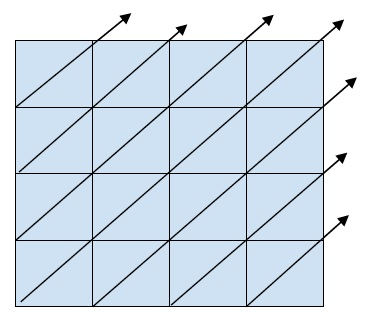# Zigzag (or diagonal) traversal of Matrix in C++

In this problem, we are given a 2D matrix. Our task is to print all the elements of the matric in a diagonal order.

Let’s take an example to understand the problem,

1    2    3
4    5    6
7    8    9

Output −

1
4    2
7    5    3
8    6
9

Let’s see the pattern that is followed while printing the matrix in a zigzag form or diagonal form.This is the way diagonal traversal works.

The number of lines in output is always dependent on the row and columns of the 2D matrix.

For a 2D matrix mat[r][c], their will be r+c-1 output lines.

## Example

Now, let’s see the solution to the program,

Live Demo

#include <iostream>
using namespace std;
#define R 5
#define C 4
int min2(int a, int b)
{ return (a < b)? a: b; }
int min3(int a, int b, int c)
{ return min2(min2(a, b), c);}
int max(int a, int b)
{ return (a > b)? a: b; }
void printDiagonalMatrix(int matrix[][C]){
for (int line=1; line<=(R + C -1); line++){
int start_col = max(0, line-R);
int count = min3(line, (C-start_col), R);
for (int j=0; j<count; j++)
cout<<matrix[min2(R, line)-j-1][start_col+j]<<"\t";
cout<<endl;
}
}
int main(){
int M[R][C] = {{1, 2, 3, 4},
{5, 6, 7, 8},
{9, 10, 11, 12},
{13, 14, 15, 16},
{17, 18, 19, 20},};
cout<<"The matrix is : \n";
for (int i=0; i< R; i++){
for (int j=0; j<C; j++)
cout<<M[i][j]<<"\t";
cout<<endl;
}
cout<<"\nZigZag (diagnoal) traversal of matrix is :\n";
printDiagonalMatrix(M);
return 0;
}

## Output

The matrix is :
1 2 3 4
5 6 7 8
9 10 11 12
13 14 15 16
17 18 19 20
ZigZag (diagonal) traversal of matrix is :
1
5 2
9 6 3
13 10 7 4
17 14 11 8
18 15 12
19 16
20